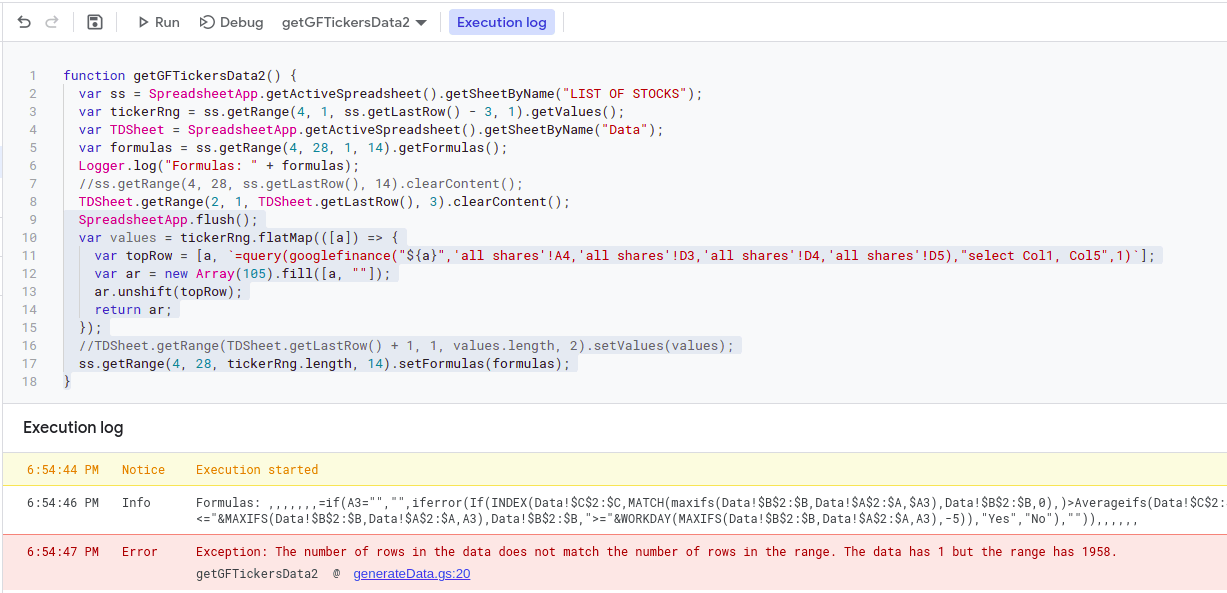# How to get formulas from a row and paste them down the range using google apps scripts?

The challenge here is to get the formulas of one row and paste them down the defined range.

The script below does big part of the job, but it fails when it comes to pasting the formulas down the rows, as per the range defined. I can't seem to extend one row of formulas into the number of formulas needed.

`tickerRng` gets the values in column A, which are all the way from 3 to row 1960.

Here's the code I'm using:

``````function getGFTickersData2() {
var ss = SpreadsheetApp.getActiveSpreadsheet().getSheetByName("LIST OF STOCKS");
var tickerRng = ss.getRange(4, 1, ss.getLastRow() - 3, 1).getValues();
var TDSheet = SpreadsheetApp.getActiveSpreadsheet().getSheetByName("Data");
var formulas = ss.getRange(4, 28, 1, 14).getFormulas();
Logger.log("Formulas: " + formulas);

ss.getRange(4, 28, ss.getLastRow(), 14).clearContent();

TDSheet.getRange(2, 1, TDSheet.getLastRow(), 3).clearContent();
SpreadsheetApp.flush();
var values = tickerRng.flatMap(([a]) => {
var topRow = [a, `=query(googlefinance("\${a}",'all shares'!A4,'all shares'!D3,'all shares'!D4,'all shares'!D5),"select Col1, Col5",1)`];
var ar = new Array(105).fill([a, ""]);
ar.unshift(topRow);
return ar;
});
TDSheet.getRange(TDSheet.getLastRow() + 1, 1, values.length, 2).setValues(values);
ss.getRange(4, 28, tickerRng.length, 14).setFormulas(formulas);
}
``````### Content Attribution

This content was originally published by Antonio Santos at Recent Questions - Stack Overflow, and is syndicated here via their RSS feed. You can read the original post over there.